# PSAT Math : How to find percentage from a fraction

## Example Questions

### Example Question #1 : Fractions And Percentage

If there are 3 boys in a class and 7 girls. What percent of the class is made up of boys?

25%

70%

3%

30%

30%

Explanation:

To solve this problem we set up a ratio of part of total. The part is the number of boys in the class and the total is the number of boys and girls in the class.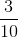now to find the percent we can multiply this fraction by 10/10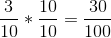From here we can see that it is 30%

### Example Question #164 : Fractions

 Marker Colors Students Blue 13 Pink 10 Orange 5 Brown 5 Green 7

The above chart shows the number of students in a class who chose each of the five marker colors available.

What percentage of the class chose a green marker?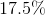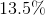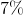Explanation:

To figure out what percentage of the class chose green markers, you must first figure out what fraction of the class chose green markers. Then, you must convert that fraction into a percentage.

Figure out the fraction:

7 students chose green markers

40 students total

Fraction of students who chose green: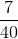To convert this fraction to a percentage, you must multiply the fraction times 100, then divide the numerator by the denominator. You multiply the fraction times 100 because in order to figure out the percent, you must figure out what the fraction means "for (per) every hundred (cent)".Multiply times 100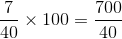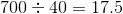Therefore, the answer is.